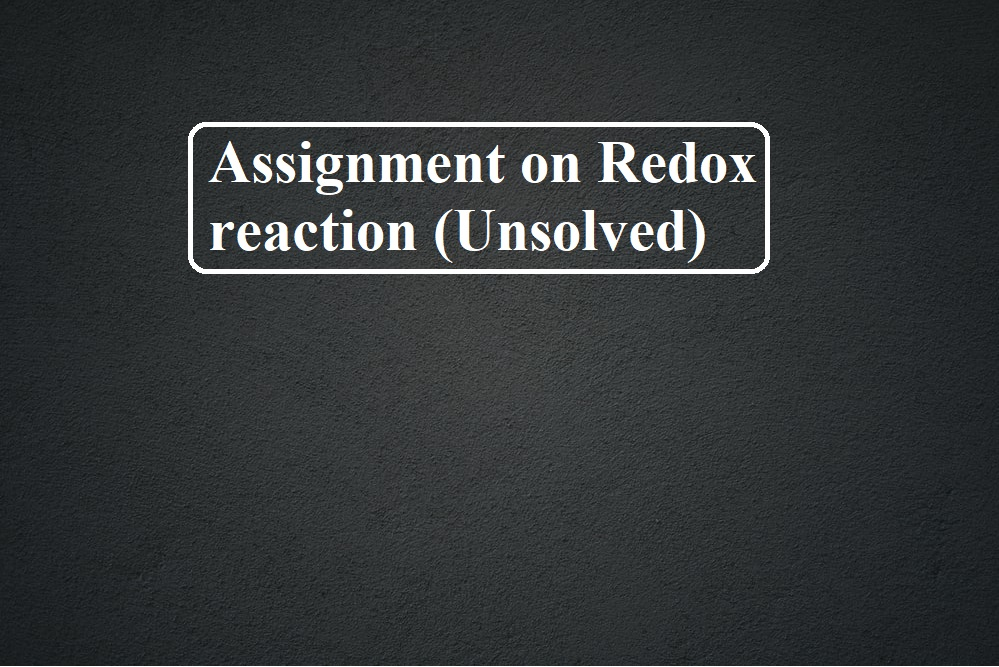# Assignment on Redox reaction (Unsolved)# Assignment on Redox reaction (Unsolved)

Assignment on Redox reaction (Unsolved)

Q.1. The colorless solution of silver nitrate slowly turns brown on adding copper chips to it because of
(a) Dissolution of Copper
(b) Oxidation of Ag+ → Ag
(c) Reduction of Cu2+ ions
(d) Oxidation of Cu atoms.

Q.2. The oxidation process involves
(a) Increase in oxidation number
(b) Decrease in oxidation number
(c) No change in oxidation number
(d) none of the above

Q.3. The oxidation number of Mn is maximum in
(a) MnO2
(b) K2MnO4
(c) Mn3O4
(d) KMnO4.

Q.4. KMnO4​reacts with oxalic acid according to the equation
2MnO4​ + 5C2​O42-​ + 16H+ → 2Mn2+​ +10CO2​ + 8H2​O, Here 20 mL of 0.1 M KMnO4​ is equivalent to
(a) 50 mL of 0.5 M C2H2O4
(b) 20 mL of 0.1 M C2H2O4
(c) 20 mL of 0.5 M C2H2O4
(d) 50 mL of 0.1 M C2H2O4

Q.5. Which of the following reactions does not involve either oxidation or reduction?
(a) VO2+ → V2O3
(b) Na → Na+
(c) CrO2-4 → Cr2O72-
(d) Zn2+ →Zn

Assignment on Redox reaction (Unsolved)

Q.6. The number of moles of KMnO4 reduced by one mole of KI in an alkaline medium is
(a) One                                   (b) Two
(c) Five                                   (d) One-fifth.

Q.7. Which of the following processes does not involve the oxidation of iron?
(a) Formation of Fe (CO)5 from Fe.
(b) Liberation of H2 from steam by iron at high temperature.
(c) Rusting of iron sheets.
(d) Decolorization of blue CuSO4 solution by iron.

Q.8. The oxidation state of Cr in Cr (CO)6 is
(a) 0                                       (b) 2
(c) 2                                       (d) 6

Q.9. Which of the following processes does not involve either oxidation or reduction?
(a) Formation of slaked lime from quick lime
(b) Heating Mercuric Oxide
(c) Formation of Manganese Chloride from Manganese oxide
(d) Formation of Zinc from Zinc blended

Q.10. The oxidation number of Cl in Cl2O7 is
(a) + 7                                                (b) + 5
(c) + 3                                                (d) – 7

Assignment on Redox reaction (Unsolved)

Q.11. A metal ion M3+ loses 3 electrons, its oxidation number will be
(a) +3                                     (b) +6
(c) 0                                       (d) -3

Q.12. The oxidation number of Sulphur in Na2S4O6 is
(a) 1.5                                    (b) 2.5
(c) 3                                       (d) 2

Q.13. In oxygen di fluoride the oxidation number of oxygen is
(a)  -2                                     (b) -1
(c) +2                                     (d) +1, -2

Q.14. Which of the following is a redox reaction?
(a) NaCl + KNO3 →NaNO3 + KCl
(b) CaC2O4 + 2HCl →CaCl2 + H2C2O4
(c) Mg (OH)2 + 2NH4Cl →MgCl2 + 2NH4OH
(d) Zn + 2AgCN →2Ag + Zn (CN)2

Q.15. Which reaction is not feasible?
(a) 2KI + Br2 →2KBr + I2
(b) 2KBr + I2 →2KI + Br2
(c) 2KBr + Cl2 →2KCl+Br2
(d) 2H2O + 2F2 →4HF+O2

ASSERTION REASONING BASED QUESTIONS

INSTRUCTION: FOR QUESTIONS NUMBER 26 TO 40.
Read the assertion and reason carefully to mark the correct option out of the options given below:
(A). If both assertion and reason are true and the reason is the correct explanation of the assertion.
(B). If both assertion and reason are true but the reason is not the correct explanation of the assertion.
(C). If the assertion is true but the reason is false.
(D). If the assertion is false but the reason is true.
(E). If the assertion and reason both are false.

Assignment on Redox reaction (Unsolved)

Q.16. Assertion: Fluorine exists only in the -1 oxidation state.
Reason: Fluorine has 2s22p5 configuration.

Q.17. Assertion: Redox reactions are called neutralization reactions.
Reason: the number of electrons gained or lost in the reaction is balanced.

Q.18. Assertion: HClO4 is a stronger acid than HClO3.
Reason: Oxidation state of Cl in HClO4 is +VII and in HClO3 is +V.

Q.19. Assertion: In a reaction Zn(s)+CuSO4(aq)→ ZnSO4(aq)+Cu(s),  Zn is a reductant but itself get oxidized.
Reason: In a redox reaction, the oxidant is reduced by accepting electrons, and the reductant is oxidized by losing electrons.

Q.20. Assertion: oxidation number of phosphorus in P4 is zero.
Reason: Phosphorus has Oxidation State zero in all its compounds.

COMPREHENSION BASED QUESTIONS

Read the given passage and answer the questions that follow: (QUESTION 41-45)

Redox reactions are reactions in which oxidation and reduction take place simultaneously. Oxidation numbers are assigned in accordance with the set of rules. Oxidation number and ion-electron methods both are used in balancing ionic equations. Redox reactions are classified as a combination, decomposition, displacement, and disproportionation reactions. The concept of redox couple and electrode processes is the basis of electrolysis and electrochemical cells.
Q.21. What are the oxidation numbers of each individual Br in Br3O8?
Q.22. If electrolysis of CuSO4 solution is carried out using Cu electrodes, what will be the reaction taking place at the anode.
Q.23. What is the oxidation number of Cr in CrO5?
Q.24. Give one example of a disproportionation reaction.
Q.25. MnO4 2– + H+ → MnO4 + MnO2 + H2O [Balance this reaction]

Assignment on Redox reaction (Unsolved)

To test your knowledge click the Quiz of chapter Chemical Bonding and Molecular Structure

https://www.sciencemotive.com/class11/quiz-chemical-bonding-and-molecular-structure/